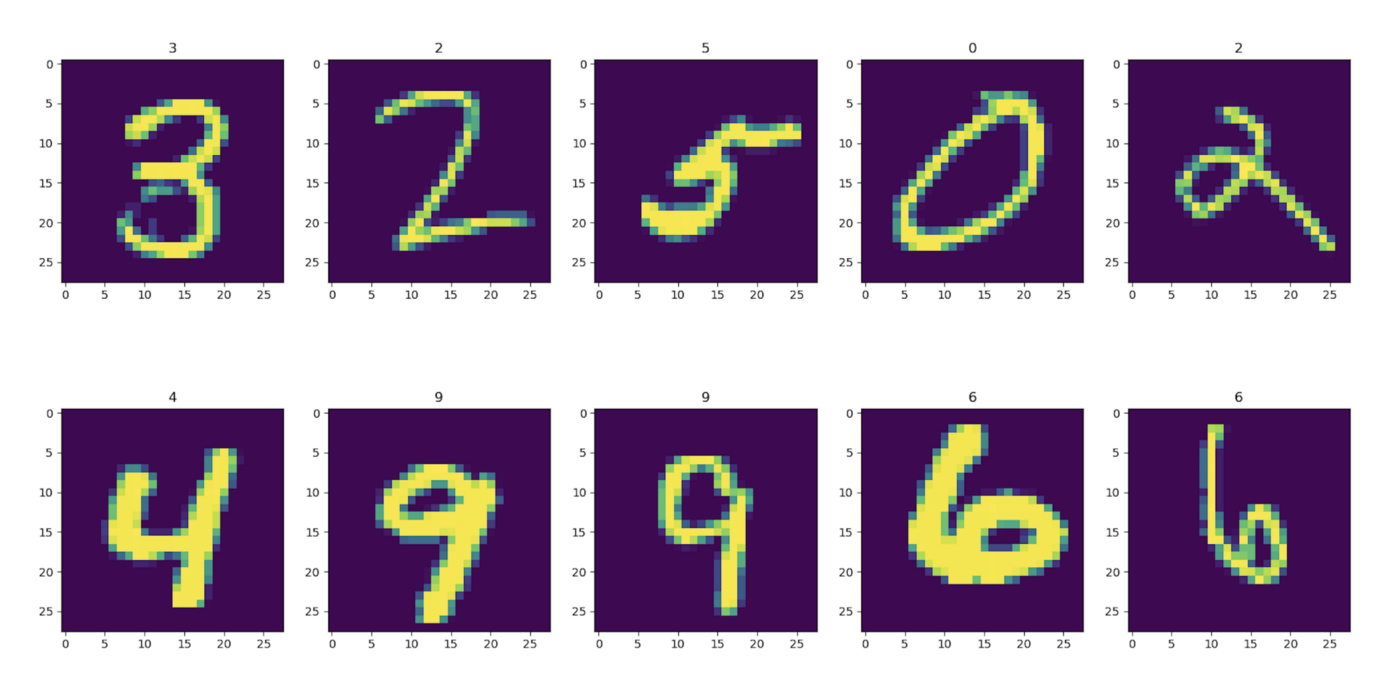# Handwritten Digit Recognition Using Machine Learning# Handwritten Digit Recognition Using Machine Learning

This is the era of digitalization, huge amount of data is being generated every second in the world. But what to do with these ? If we are able to draw some insights from the data then this will be beneficial to all of us and that’s where Machine Learning comes into picture.

# What is Machine Learning ?

Machine learning(ML) is the science of getting computers to act without being explicitly programmed .In layman’s term, Machine Learning is the process of teaching computers to learn and make better predictions of future events from past experiences as we humans do. So, how computers learn ? Computers learn with the help of some set of rules called Algorithms, we feed the algorithms with data and it figures out the patterns existing in it. And with the help of these patterns computers predict the result of the future events. Why Machine Learning is important ? Starting from YouTube video recommendation to self-driving car Machine Learning is everywhere.

In this article we are going to Recognize handwritten digits from the digits dataset of sklearn.dataset library and test the hypothesis that, “Does the digits dataset of scikit-learn library predicts the digit accurately 95% of the times or not”.

# What is Handwritten Digit Recognition ?

The handwritten digit recognition is the ability of computers to recognize human handwritten digits. It is a hard task for computers because handwritten digits can be of any size, shape and there are also some similar digits like 3 and 8, 1 and 7 etc.

As we are asked to test the hypothesis that whether the digits dataset predicts digits accurately 95% of the time or not, so we are going to take 2 different algorithms if for both the algorithms the dataset gives accurate predictions then we will accept the Null Hypothesis otherwise reject it.

Let’s import all the necessary libraries as well as the dataset. Here the dataset is loaded into df_1 and by using dir() we are able to get the attributes of the dataset namely DESCR, data, images, target, target_ names.

Each sample in this dataset is an 8×8 image represents a handwritten digit, each pixel is represented by an integer in the range 0 to 16.The data attribute contains the images as flattened array of 64 pixels .The target attribute contains value of the digit ranging from 0 to 9.So it’s a classification problem. With the len() function we are got the length of the dataset to be 1797.

Checking out some random images and plotting them in a figure.

First we have applied the SVM(Support Vector Machine) algorithm, to choose best parameters first we have applied the GridSearchCV then found out the best parameters from that using best_params_ method and with those parameters we have trained the data. Out of 1797 we have chosen 1257 sample for training and rest for testing(70:30).

Then we plotted the confusion matrix and found out the accuracy score and the accuracy was around 95.19%.

The same method was applied for DecisionTreeClassifier but in that case the accuracy was around 77%.

Then we tried out the DecisionTreeClassifier without GridSearchCV but in this case the accuracy was quite low also around 79%.

So from the above discussions we can conclude that not all the time the dataset gives accuracy greater than 95%; it depends upon the algorithm with which we are trying to predict as well as the parameters of that algorithm also. So we are going to reject the Null Hypothesis and accept the Alternate one.

“I am thankful to mentors at https://internship.suvenconsultants.com for providing awesome problem statements and giving many of us a Coding Internship Experience. Thank you www.suvenconsultants.com”

The code for the project can be found out at GitHub.